What is a Goal Seek

Goal Seek is a formula which is used for Reverse Calculation

It can only be used if some formula or links have been used while passing entries

How to do?

Data-->What-if Analysis--->Goal Seek

For Excel 2003: Tools --> Goal Seek

Set Cell —Select Cell which has old value (This should have formulas)

To Value —Put New Value(This should have new value)

By Changing -Select Cell where Answer Required (This should not have a formula)

Example 1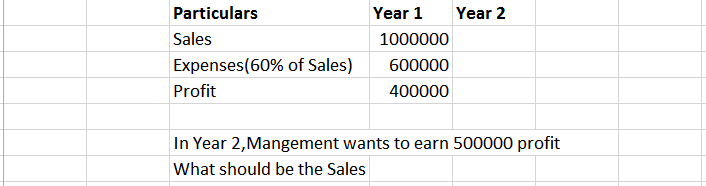(For Excel 97, 2003, 2007)

Example 2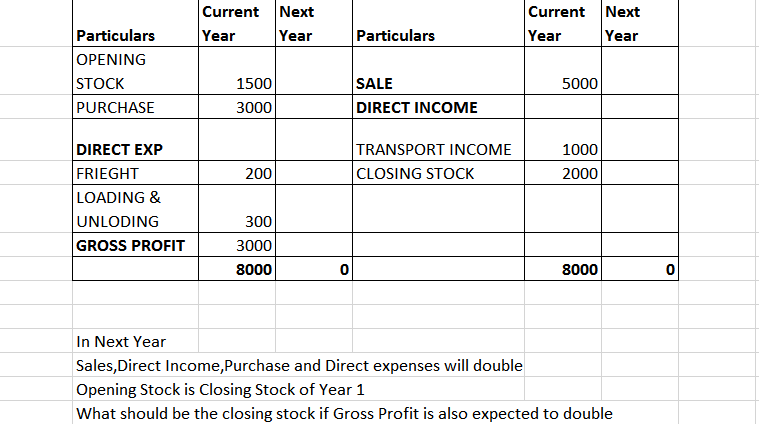Example 3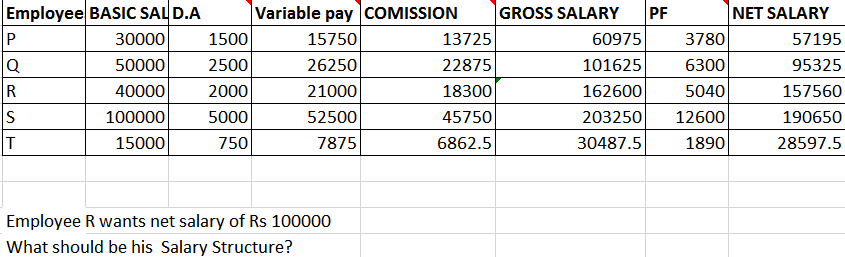Example 4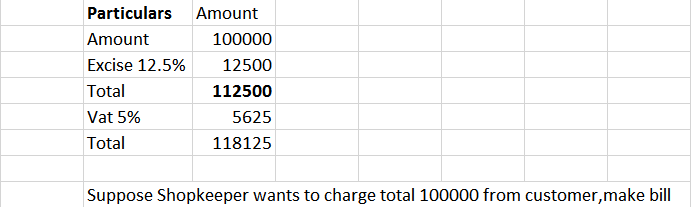Example 5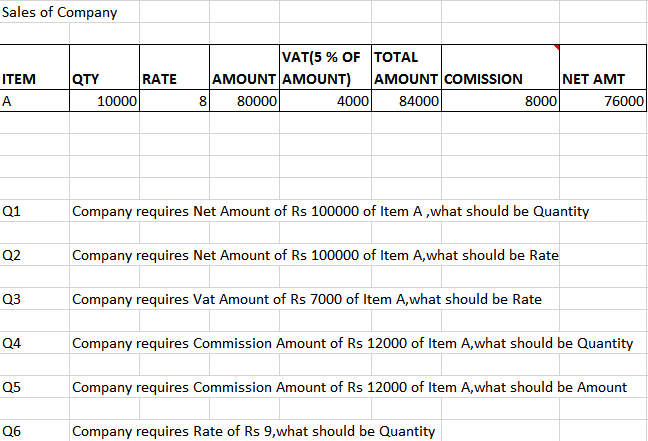1. Excel
Accounts Tax
Excel